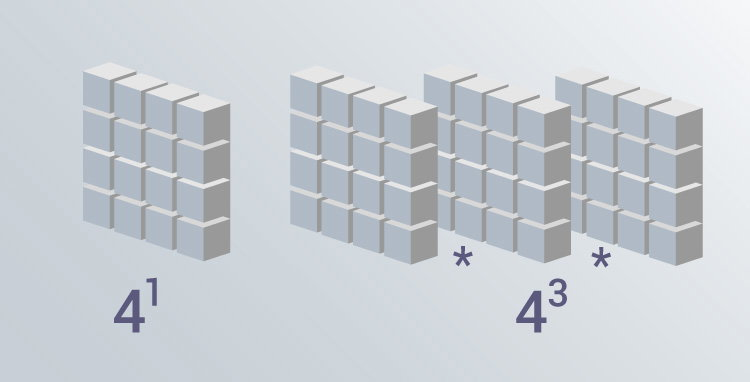# C Program to Calculate the Power of a Number

In this example, you will learn to calculate the power of a number.To understand this example, you should have the knowledge of the following C programming topics:

The program below takes two integers from the user (a base number and an exponent) and calculates the power.

For example: In the case of 23

• 2 is the base number
• 3 is the exponent
• And, the power is equal to `2*2*2`

## Power of a Number Using the while Loop

``````#include <stdio.h>
int main() {
int base, exp;
long long result = 1;
printf("Enter a base number: ");
scanf("%d", &base);
printf("Enter an exponent: ");
scanf("%d", &exp);

while (exp != 0) {
result *= base;
--exp;
}
return 0;
}
``````

Output

```Enter a base number: 3
Enter an exponent: 4
```

The above technique works only if the exponent is a positive integer.

If you need to find the power of a number with any real number as an exponent, you can use the `pow()` function.

## Power Using pow() Function

``````#include <math.h>
#include <stdio.h>

int main() {
double base, exp, result;
printf("Enter a base number: ");
scanf("%lf", &base);
printf("Enter an exponent: ");
scanf("%lf", &exp);

// calculates the power
result = pow(base, exp);

printf("%.1lf^%.1lf = %.2lf", base, exp, result);
return 0;
}
``````

Output

```Enter a base number: 2.3
Enter an exponent: 4.5
2.3^4.5 = 42.44
```